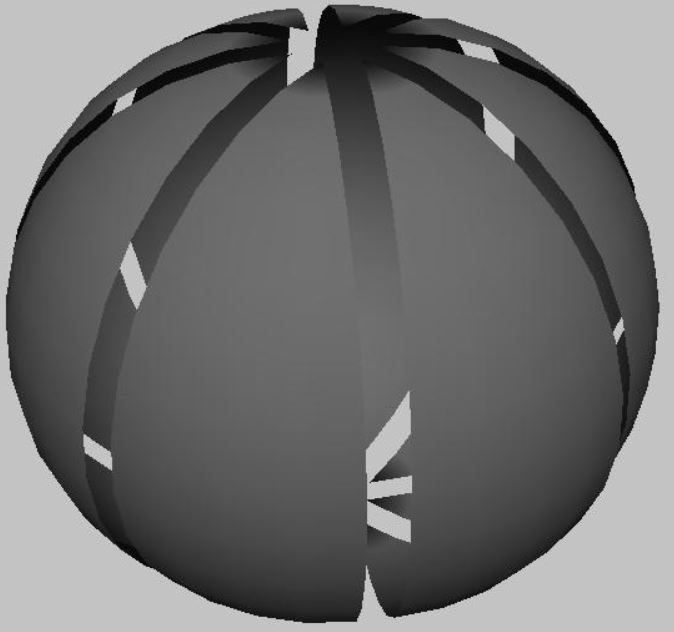# nLab G-representation spheres are G-CW-complexes

Contents

### Context

#### Representation theory

representation theory

geometric representation theory

# Contents

## Statement

###### Proposition

(G-representation spheres are G-CW-complexes)

For $G$ a compact Lie group (e.g. a finite group) and $V \in RO(G)$ a finite-dimensional orthogonal $G$-linear representation, the representation sphere $S^V$ admits the structure of a G-CW-complex.

###### Proof

Observe that we have a $G$-equivariant homeomorphism between the representation sphere of $V$ and the unit sphere in $\mathbb{R} \oplus V$, where $\mathbb{R}$ is the 1-dimensional trivial representation (this Prop.)

(1)$S^V \;\simeq\; S(\mathbb{R} \oplus V) \,.$

It is thus sufficient to show that unit spheres in orthogonal representations admit G-CW-complex structure.

This in turn follows as soon as there is a $G$-equivariant triangulation of $S(\mathbb{R}\oplus V)$, hence a triangulation with the property that the $G$-action restricts to a bijection on its sets of $k$-dimensional cells, for each $k$. Because then if $G/H$ is an orbit of this $G$-action on the set of $k$-cells, we have a cell $G/H \times D^k$ of an induced G-CW-complex.

Since the unit spheres in (1) are smooth manifolds with smooth $G$-action, the existence of such $G$-equivariant triangulations follows for general compact Lie groups $G$ from the equivariant triangulation theorem (Illman 83).

More explicitly, in the case that $G$ is a finite group such an equivariant triangulation may be constructed as follows:

Let $\{b_1, b_2, \cdots, b_{n+1}\}$ be an orthonormal basis of $\mathbb{R} \oplus V$. Take then as vertices of the triangulation all the distinct points $\pm g(b_i) \in \mathbb{R} \oplus V$, and as edges the geodesics (great circle segments) between nearest neighbours of these points, etc.

## Examples

###### Example

($\mathbb{Z}_n$-CW-decomposition of 2-sphere with rotation action)

For $n \in \mathbb{N}$, $n \geq 2$, let $\mathbb{Z}_n \hookrightarrow SO(2)$ be the cyclic group acting by rotations on the plane $\mathbb{R}^2$. Writing $\mathbb{R}^2_{rot_n}$ for the corresponding representation, its representation sphere $S^{\mathbb{R}^2_{rot_n}}$ has a G-CW-complex structure as follows:1. The vertices are the two fixed point poles $(G/G) \times \{0\} = \{0\}$ and $(G/G) \times \{\infty\} = \{\infty\}$;

2. the edges are $n$ great circle arcs obtained from any one such arc from $0$ to $\infty$ together with all its images under $G$, hence together a free $G$-orbit $(G/1) \times D^1 = G \times D^1$ of 1-cells;

3. the faces are the $n$ bigons between each such arc and the next one, hence together a free orbit $(G/1) \times D^2 = G \times D^2$ of 2-cells.

The graphics on the right illustrates this cell decomposition for $n = 8$:

graphics grabbed from here

• Sören Illman, Smooth equivariant triangulations ofG-manifolds for $G$ a finite group, Math. Ann. (1978) 233: 199 (doi:10.1007/BF01405351)

• Sören Illman, The Equivariant Triangulation Theorem for Actions of Compact Lie Groups, Mathematische Annalen (1983) Volume: 262, page 487-502 (dml:163720)